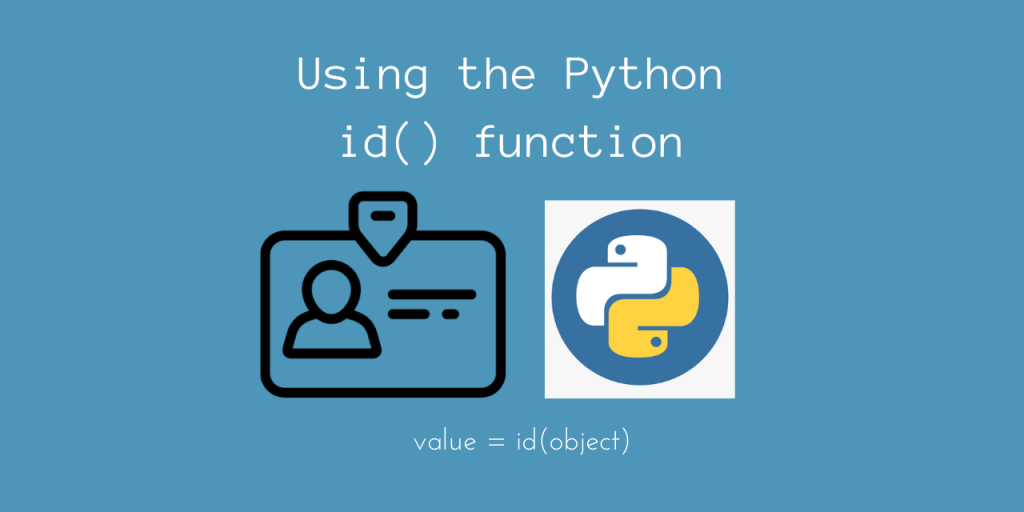# Using the id() function in PythonHello everyone! In today’s article, we’ll take a look at the id() function in Python.

The id() function returns the identity of any Python object. This will return an integer identification number for different objects.

The underlying CPython implementation uses the `id()` function as the address of the object, in memory.

Let’s understand this a bit more, using some examples.

## Basic Syntax of id() function in Python

This function takes any Python object – be it an integer, float, string, list, Class, function, lambda, etc, and returns an integer id.

```val = id(object)
```

## Using id() in Python

The id of an object is useful for Python to cache the values of those variables. This mechanism of retrieving cached values using `id()` makes Python perform better!

This also helps in cases where multiple variables refer to the same object.

```a = 1233.45
b = a

print(id(a))
print(id(b))
```

Output

```2775655780976
2775655780976
```

In this case, it would be easier for Python to keep track of the referenced object, so the id() of a will be the same as that of b.

Now let’s try to use this on some simple Python objects.

```print(id(103)) # Int

print(id(104))

print(id(10.25)) # Float

print(id([1, 2, 3])) # List

print(id(lambda x: x * x)) # Lambda
```

Output

```1658621232
1658621264
2775655780976
2775665230232
2775665206344
2775656111776
```

As you can observe, the id() values of integers may vary based on their value, and represent the size of the integer object in bytes. The id() function returns a byte address, not a bit address.

So Python stores the list of all the integers in sequential blocks, which are equally spaced. Makes sense?

### Now, let’s test id() on strings

```# strings
s1 = 'ABC'
s2 = 'ABC'
print(id(s1))
print(id(s2))
```

Output

```2775656418080
2775656418080
```

As you can observe, Python does indeed cache strings so as to preserve memory!

Remember that caching can work only on immutable Python objects, like integer, string, and floats. Tuples, Lists, etc are mutable objects, so caching will not work here!

To prove this, let’s check the id’s of two lists having the same elements:

```> l1 = [1, 2, 3, 4]
> l2 = [1, 2, 3 ,4]
> id(l1)
2775665206344
> id(l2)
2775665185224
```

Here, since the lists are mutable, there isn’t any caching involved.

## Using id() on a Custom Object

We can also use the id() function on custom objects.

Let’s take a simple example:

```class Student():
def __init__(self, name, id):
self.name = name
self.id = id

s = Student('Amit', 10)
t = Student('Rahul', 20)

print(id(s))

print(id(t))
```

Output

```2775665179336
2775665179448
```

This refers to the memory address where the objects are stored, which are obviously different for the two instances!

## Conclusion

In this article, we learned about using the id() function in Python. This represents the underlying memory address of the Python object, which is useful in caching immutable objects.

## References

• JournalDev article on Python id() function